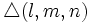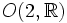# Triangle group

This article defines a family of groups whose members are parametrized by tuples of natural numbers. In other words, for every tuple of natural numbers, there is a unique corresponding group (upto isomorphism) in that family

## Definition

### In terms of presentations

Let$(l,m,n)$ be an (unordered) tuple of natural numbers. The triangle group$\triangle(l,m,n)$ is defined by the following presentation:$\langle a,b,c \mid a^2 = b^2 = c^2 = (ab)^l = (bc)^m = (ca)^n = 1 \rangle$

Here$l,m,n$ are integers greater than or equal to 2.

Equivalently, it is a Coxeter group on three generators, where the non-diagonal entries are$l,m,n$.

### Geometric description

The triangle group with parameters$(l,m,n)$ is the group of isometries in a suitable simply connected Riemannian surface (the Euclidean plane, sphere or hyperbolic plane) generated by reflections about the sides of a triangle with angles$\pi/l, \pi/m, \pi/n$.

Note that sometimes the term triangle group is used for von Dyck group which is actually the subgroup of index two in the triangle group comprising all the elements expressible using words of even length (in other words, all the orientation-preserving transformations).

## The three types

### Spherical triangle groups

This corresponds to the case:$\frac{1}{l} + \frac{1}{m} + \frac{1}{n} > 1$.

to which the solutions are$(n,n,1)$,$(n,2,2)$,$(3,3,2)$,$(4,3,2)$, and$(5,3,2)$.

The model for all of these is spherical geometry, i.e., the unit sphere. Since the isometry group of this is$O(3,\R)$, these are all finite subgroups of$O(3,\R)$. In fact, they are the only finite subgroups of$O(3,\R)$ that contain orientation-reversing transformations.

### Euclidean triangle groups

This corresponds to the case:$\frac{1}{l} + \frac{1}{m} + \frac{1}{n} = 1$,

with solutions$(3,3,3)$ and$(4,4,2)$, corresponding to the equilateral triangle and right isosceles triangle in the Euclidean plane. Both of these give rise to wallpaper groups, neither of which is finite.

### Hyperbolic triangle groups

This corresponds to the case:$\frac{1}{l} + \frac{1}{m} + \frac{1}{n} < 1$,

This has infinitely many solutions.

## Particular cases

Smallest parameter Middle parameter Largest parameter Common name for group Group order Symmetry object
1$n$$n$ Dihedral group$2n$ Regular polygon, symmetries in$O(2,\R)$
2 3 3 Symmetric group:S4$24$ Regular tetrahedron, symmetries in$O(3,\R)$
2 3 4 Cube group$48$ Cube or octahedron, symmetries in$O(3,\R)$
2 3 5 Direct product of alternating group:A5 and cyclic group:Z2$120$ Icosahedron or dodecahedron, symmetries in$O(3,\R)$